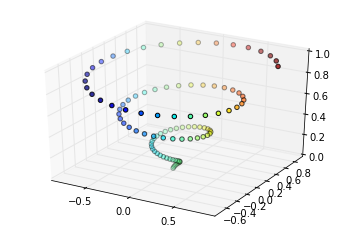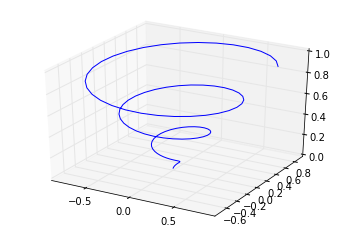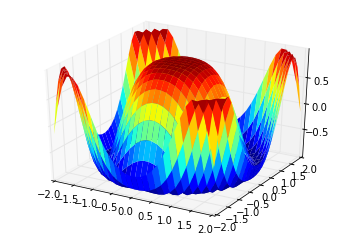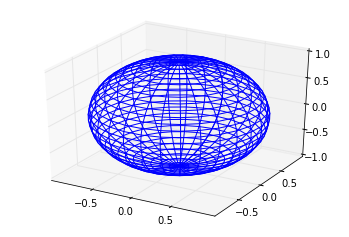# 5. 3D Plotting¶

Use the “v2” files in older versions of IPython, e.g. 0.12

# Matplotlib Tutorial: 5. 3D plotting

The 3D plotting toolkit introduced in matplotlib version 1.0 can lead to some very nice plots. We'll explore a few of the options here: for more examples, the matplotlib tutorial is a great resource.

Again we'll use inline plotting, though it can be useful to skip the "inline" backend to allow interactive manipulation of the plots.

In :
%pylab inline

Welcome to pylab, a matplotlib-based Python environment [backend: module://IPython.zmq.pylab.backend_inline].

In :
# This is the 3D plotting toolkit
from mpl_toolkits.mplot3d import Axes3D


Just as before when we created a 2D axes and called several plotting methods on it, here we'll create some 3D axes objects and call 3D plotting routines. Below are several examples.

## 3D scatter Plot

The 3D scatter plot takes all the same keyword parameters as the 2D scatter plot, so its use should be familiar. To create a 3D axes, we need to pass the argument projection='3d'.

In :
fig = plt.figure()
ax = plt.axes(projection='3d')

z = np.linspace(0, 1, 100)
x = z * np.sin(20 * z)
y = z * np.cos(20 * z)

c = x + y

ax.scatter(x, y, z, c=c)

Out :
<mpl_toolkits.mplot3d.art3d.Patch3DCollection at 0x9bce74c>## 3D Line Plot

Like the 2D and 3D scatter command, the 2D and 3D plot command have the same argument structure. Thus plotting a 3D line plot is straightforward:

In :
fig = plt.figure()
ax = plt.axes(projection='3d')

ax.plot(x, y, z, '-b')

Out :
[<mpl_toolkits.mplot3d.art3d.Line3D at 0x9b7daac>]## Surface Plot

Surface plots are connected plots of x, y, and z coordinates. They can be used to make some pretty interesting shapes:

In :
x = np.outer(np.linspace(-2, 2, 30), np.ones(30))
y = x.copy().T
z = np.cos(x ** 2 + y ** 2)

fig = plt.figure()
ax = plt.axes(projection='3d')

ax.plot_surface(x, y, z, cmap=plt.cm.jet, rstride=1, cstride=1, linewidth=0)

Out :
<mpl_toolkits.mplot3d.art3d.Poly3DCollection at 0x9f4570c>## Wire-frame plot

Wire-frame plots draw lines between nearby points. They can be displayed using the plot_wireframe method. Here we'll plot a parametrized sphere:

In :
u = np.linspace(0, np.pi, 30)
v = np.linspace(0, 2 * np.pi, 30)

x = np.outer(np.sin(u), np.sin(v))
y = np.outer(np.sin(u), np.cos(v))
z = np.outer(np.cos(u), np.ones_like(v))

fig = plt.figure()
ax = plt.axes(projection='3d')

ax.plot_wireframe(x, y, z)

Out :
<mpl_toolkits.mplot3d.art3d.Line3DCollection at 0x9f2162c>